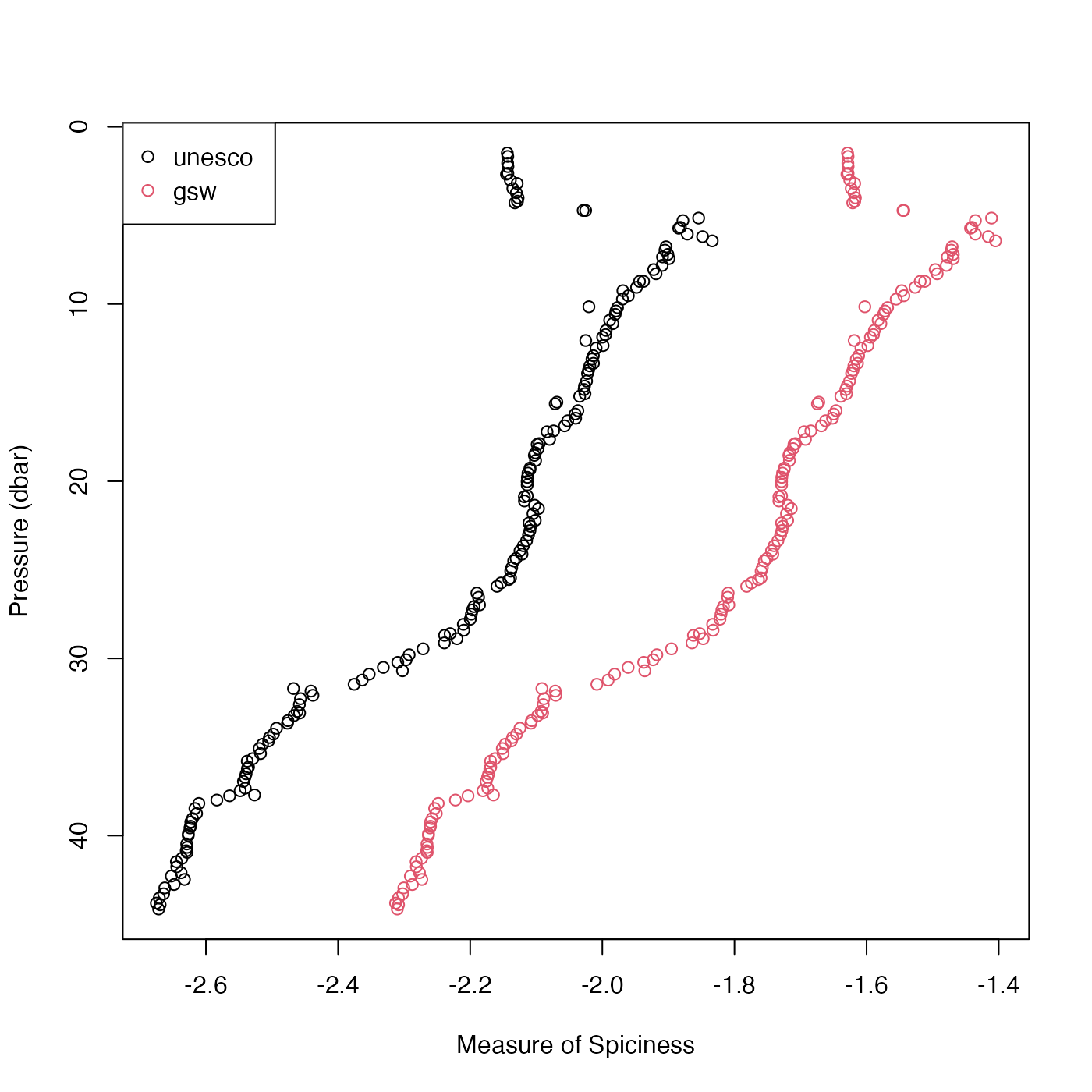Compute seawater "spice", also called "spiciness" (a variable orthogonal to density in TS space), in either of two formulations, depending on the value of the eos argument. If eos="unesco" then Flament's (reference 1) formulation is used. If eos="gsw" then the Gibbs SeaWater formulation for "spiciness0" is used (see reference 2).

swSpice(
salinity,
temperature = NULL,
pressure = NULL,
longitude = NULL,
latitude = NULL,
eos = getOption("oceEOS", default = "gsw")
)

## Arguments

salinity

either salinity (PSU) (in which case temperature and pressure must be provided) or a ctd object (in which case salinity, temperature and pressure are determined from the object, and must not be provided in the argument list).

temperature

in-situ temperature ($$^\circ$$C) on the ITS-90 scale; see “Temperature units” in the documentation for swRho().

pressure

Seawater pressure (dbar) (only used if eos is "gsw"); see “Details”..

longitude

longitude of observation (only used if eos is "gsw"; see “Details”).

latitude

latitude of observation (only used if eos is "gsw"; see “Details”).

eos

Character value specifying the equation of state, either "unesco" (for the Flament formulation, although this is not actually part of UNESCO) or "gsw" for the Gibbs SeaWater formulation.

## Value

Flament-formulated spice $$kg/m^3$$ if eos is "unesco"

or surface-referenced GSW spiciness0 $$kg/m^3$$ if eos is "gsw", the latter provided by gsw::gsw_spiciness0(), and hence aimed at application within the top half-kilometre of the ocean.

## Details

If the first argument is a ctd object, then salinity, temperature and pressure values are extracted from it, and used for the calculation. (For the eos="gsw" case, longitude and latitude are also extracted, because these are required for the formulation of spiciness0.

Roughly speaking, seawater with a high spiciness is relatively warm and salty compared with less spicy water. Another interpretation is that spice is a variable measuring distance orthogonal to isopycnal lines on TS diagrams (if the diagrams are scaled to make the isopycnals run at 45 degrees). Note that pressure, longitude and latitude are all ignored in the Flament definition.

Other functions that calculate seawater properties: T68fromT90(), T90fromT48(), T90fromT68(), computableWaterProperties(), locationForGsw(), swAbsoluteSalinity(), swAlphaOverBeta(), swAlpha(), swBeta(), swCSTp(), swConservativeTemperature(), swDepth(), swDynamicHeight(), swLapseRate(), swN2(), swPressure(), swRho(), swRrho(), swSCTp(), swSR(), swSTrho(), swSigma0(), swSigma1(), swSigma2(), swSigma3(), swSigma4(), swSigmaTheta(), swSigmaT(), swSigma(), swSoundAbsorption(), swSoundSpeed(), swSpecificHeat(), swSstar(), swTFreeze(), swTSrho(), swThermalConductivity(), swTheta(), swViscosity(), swZ()

## Author

Dan Kelley coded this, merely an interface to the code described by references 1 and 2.

## Examples

# Contrast the two formulations.
library(oce)
data(ctd)
p <- ctd[["pressure"]]
plot(swSpice(ctd, eos="unesco"), p,
xlim=c(-2.7, -1.5), ylim=rev(range(p)),
xlab="Spice", ylab="Pressure (dbar)")
points(swSpice(ctd, eos="gsw"), p,col=2)
mtext("black=unesco, red=gsw")